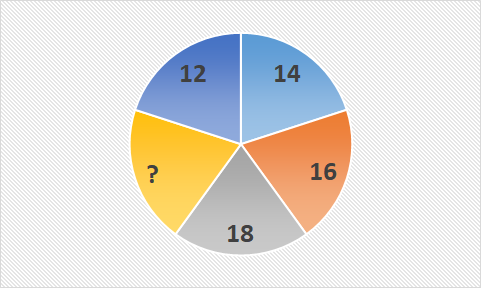# Examples of Number Patterns in Mathematics

Examples of Number Patterns in Mathematics, deals with various concepts which are as under:-

• Number Patterns
• Increasing Number Patterns
• Decreasing Number Patterns

### Examples of Number Patterns in Mathematics – Number Patterns

Example 1

Find the missing number in the given figure,assuming 12 as starting point ?Explanation:

In the given figure we can observe, that the number on right of any given number is 2 more than the previous number For example, number after 12 is (12+2) = 14
number after 14 is (14+2) = 16 and so on.

Therefore to get the missing number we need to add 2 to the previous number So, 18 + 2 = 20

So the missing number is 20

### Examples of Number Patterns in Mathematics – Increasing Number Patterns

Example 2

Find the next term of the series .
8 , 11 , 14 , 17 , ___

Explanation:

In the given series
8 , 11 , 14 , 17 , ___
8 + 3 = 11
11 + 3 = 14
14 + 3 = 17
We have observed that, every number is 3 more than the previous number. Therefore to get the next number of the series we need to add 3 to the previous number
So, we can write the next numbers as
17 + 3 = 20
Therefore the next term of the series is 20

Example 3

Find the next term of the series
3 , 6 , 10 , 15 , ___

Explanation:

In the given series
3 , 6 , 10 , 15 , ___
3 + 3 = 6
6 + 4 = 10
10 + 5 = 15
We have observed that, every number is increased by 3 , 4 , 5 and so on
Therefore to get the next number of the series we need to add 6 to the previous number
So,
15 + 6 = 21
Hence the next number of the series is 21

Example 4

Find the next term of the series
6 , 12 , 24 , 48 , ____

Explanation:

In the given series
6 , 12 , 24 , 48 , ____
6 x 2 = 12
12 x 2 = 24
24 x 2 = 48
We have observed that, every number is 2 times the previous number. Therefore to get the next number of the series we need to multiply the previous number by 2
So, we can write the next numbers as
48 x 2 = 96
Therefore the next term of the series is 96

### Examples of Number Patterns in Mathematics – Decreasing Number Patterns

Example 5

In the given series Find the next term of the series
27 , 26 , 25 , 24 , ___

Explanation:

27 , 26 , 25 , 24 , ___
27 – 1 = 26
26 – 1 = 25
25 – 1 = 24
We have observed that, every number is 1 less than the previous number. Therefore to get the next number of the series we need to subtract 1 from the previous number
So, we can write the next numbers as
24 – 1 = 23
Therefore the next term of the series is 23

Example 6

Find the next term of the series
12 , 11 , 9 , 6 , ____

Explanation:

In the given series
12 , 11 , 9 , 6 , ____
12 – 1 = 11
11 – 2 = 9
9 – 3 = 6
Since, each number is decreased by 1 , 2 , 3 and so on to its previous number
Therefore to get the missing number, we need to subtract 4 from the previous number
So,
6 – 4 = 2
Hence the next number of the series is 2